## Fractal Dimension

Lines are $1$-dimensional, areas are $2$-dimensional, and in the previous challenge we found that a coastline is somewhere in between. With all their twists and turns and rough edges, coastlines fill more space than a line, but not as much as an area.

A coastline is a naturally-occurring example of a fractal — a hard-to-define kind of mathematical object which is typically of a surprising dimension. Keep reading to learn more about fractals with a specific example, or jump right to the fractal in today’s challenge.

One defining feature of fractals is self-similarity: if you zoom in on a fractal, you’ll see that it is made of pieces that are similar to its overall shape.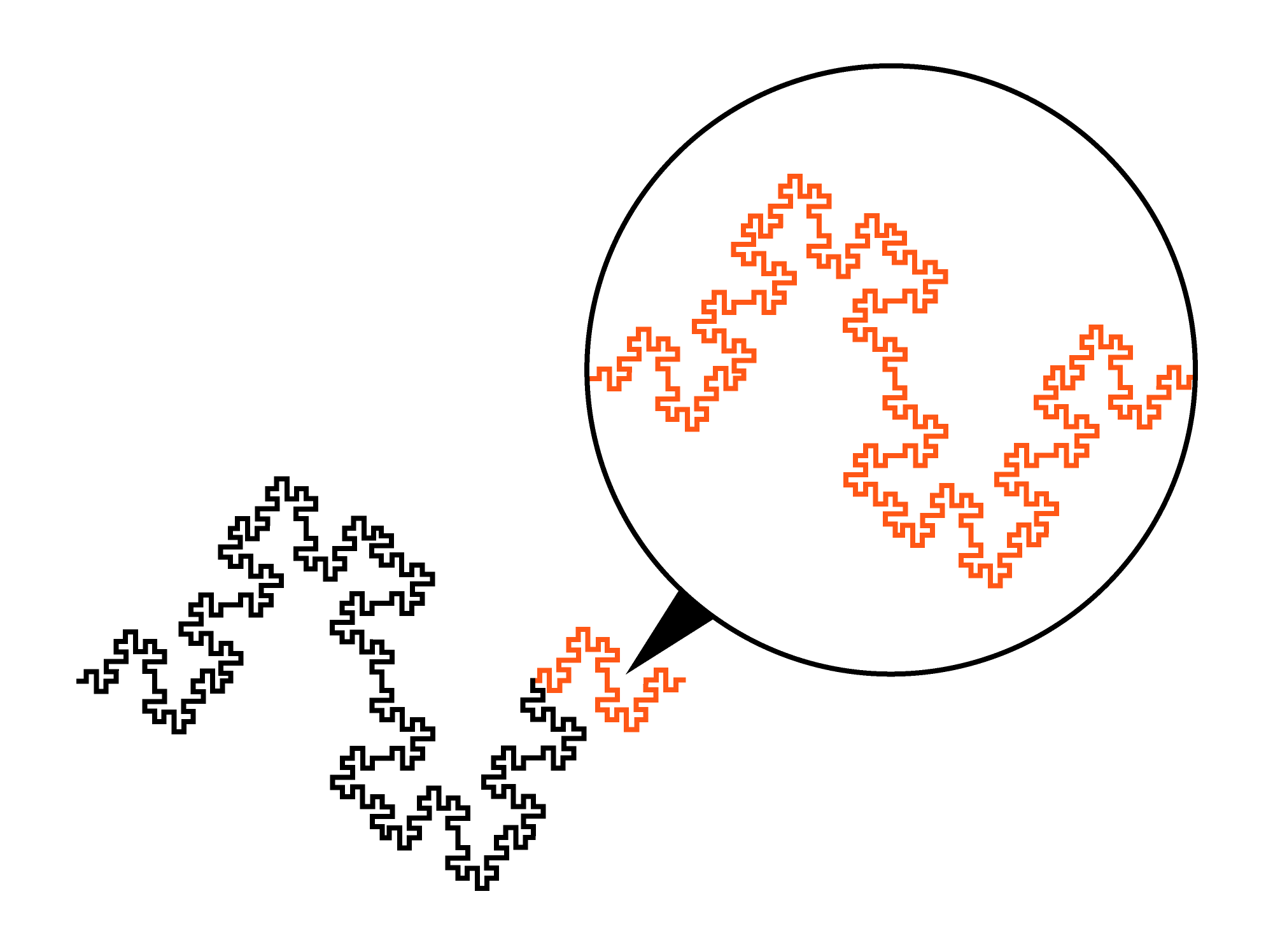There are a number of ways to define a given fractal; one way that captures their self-similarity is an iterative procedure with “initiators” and “generators.” Here’s a method that will create the fractal above: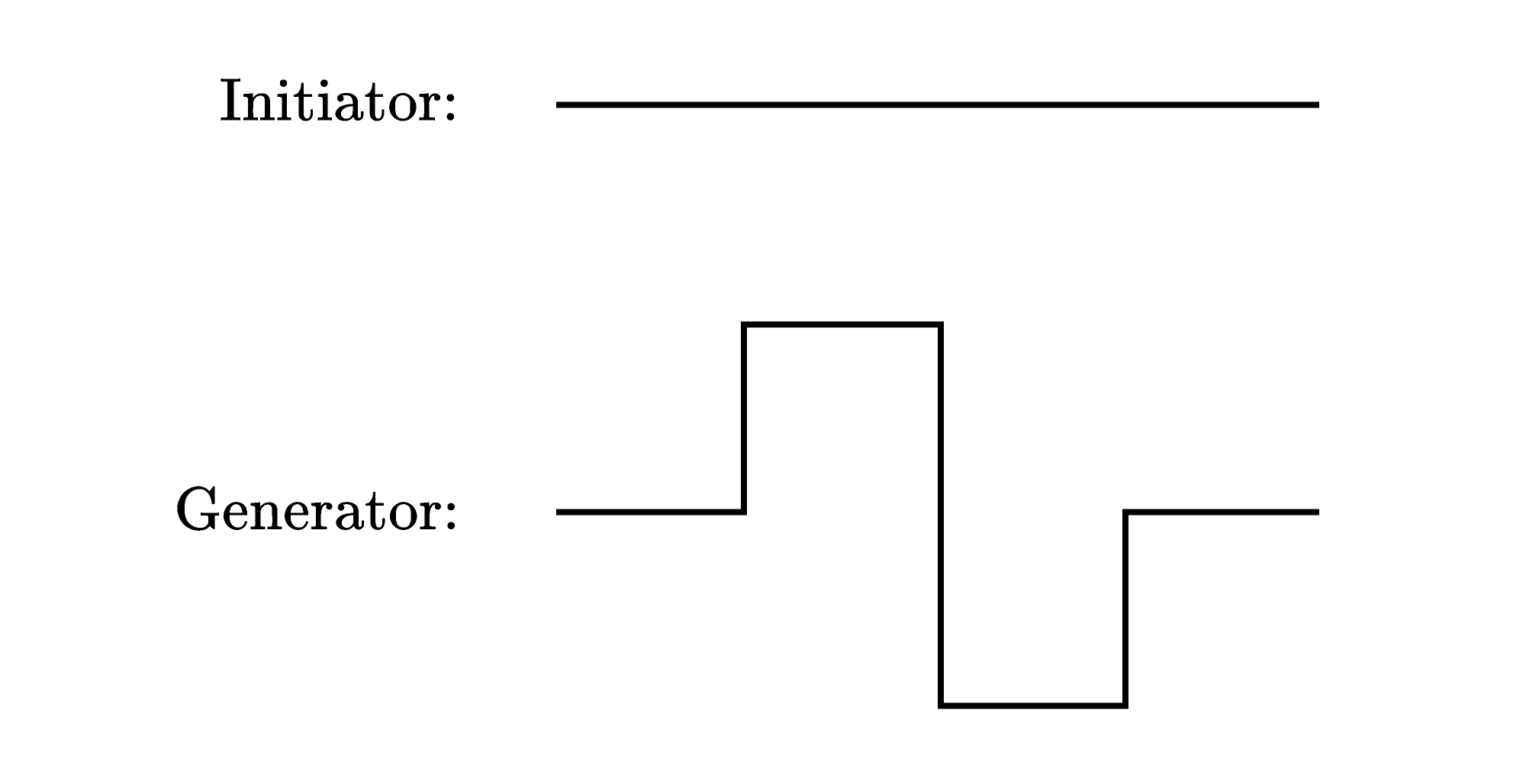Initiator and Generator

This initiator is a line segment, and the generator is an arrangement of eight horizontal and vertical line segments, all the same length (in the middle, two vertical line segments are stacked). From the left end to the right end, the generator is the same length as the initiator.

To go from one stage to the next, replace every copy of the initiator with a copy of the generator, scaled down and rotated as necessary. The initiator and the generator are the first two stages, and the third looks like this: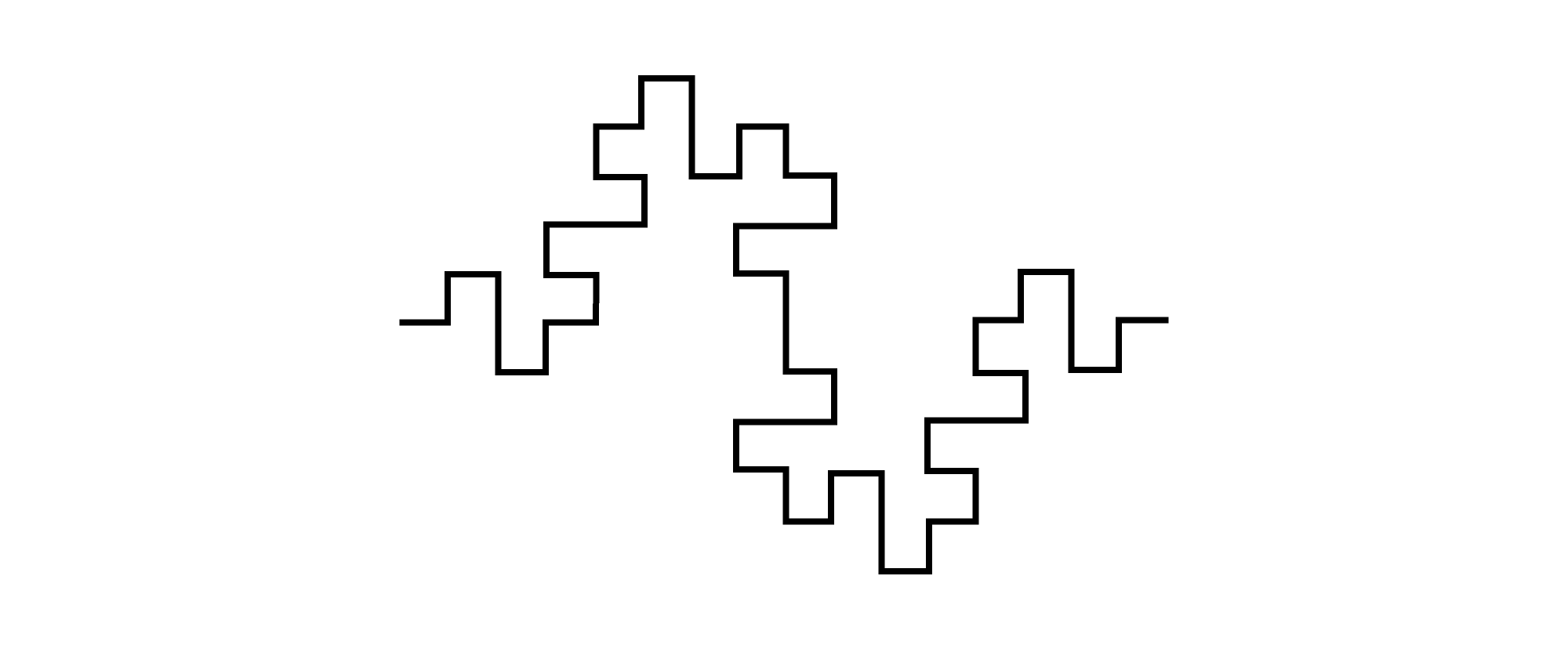Stage Three

Since the initiator is a line segment, to make the third stage, each line segment in the generator is replaced with a scaled-down and rotated copy of the generator. The generator takes up the same amount of horizontal space as the initiator, and its horizontal length is made up of four equal-length line segments (shown in red below):So, to be able to replace a line segment with a generator, each line segment of the generator must be scaled down by a factor of $S=4.$ And, as stated above, we replace each line segment with $C=8$ scaled-down copies of itself.

We see that, from one stage to the next, as the scale is reduced, this fractal grows in complexity very quickly. This is similar to a coastline, where using smaller units of measurement reveals more of the twists and turns and rough edges. So maybe their dimensions are similar?

First, let’s rephrase our dimension equation to be more clearly applicable to fractals defined with initiators and generators:

The dimension, $D,$ of an iteratively defined fractal satisfies the equation $C = S^D$ if, to go from one stage to the next, we replace each initiator with $C$ copies of itself, which have been scaled down by a factor of $S.$

Then with $C=8$ and $S=4,$ the dimension of our fractal satisfies $8=4^D.$ That means $D=1.5.$ Just like a coastline, this fractal’s dimension is between $1$ and $2.$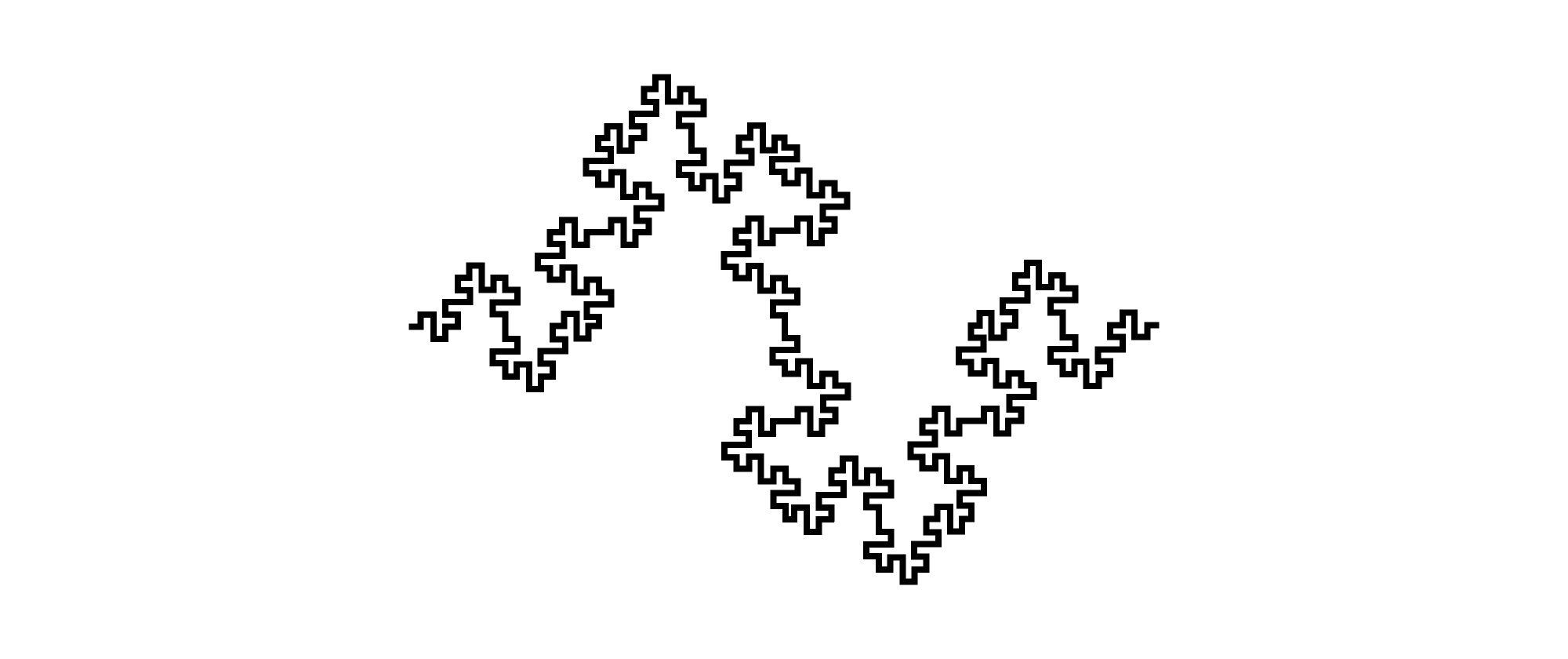Stage Four

Now it’s your turn to calculate the dimension of a different fractal.

# Today's Challenge

A fractal can be defined by an iterative procedure of "initiators" and "generators." Consider the following: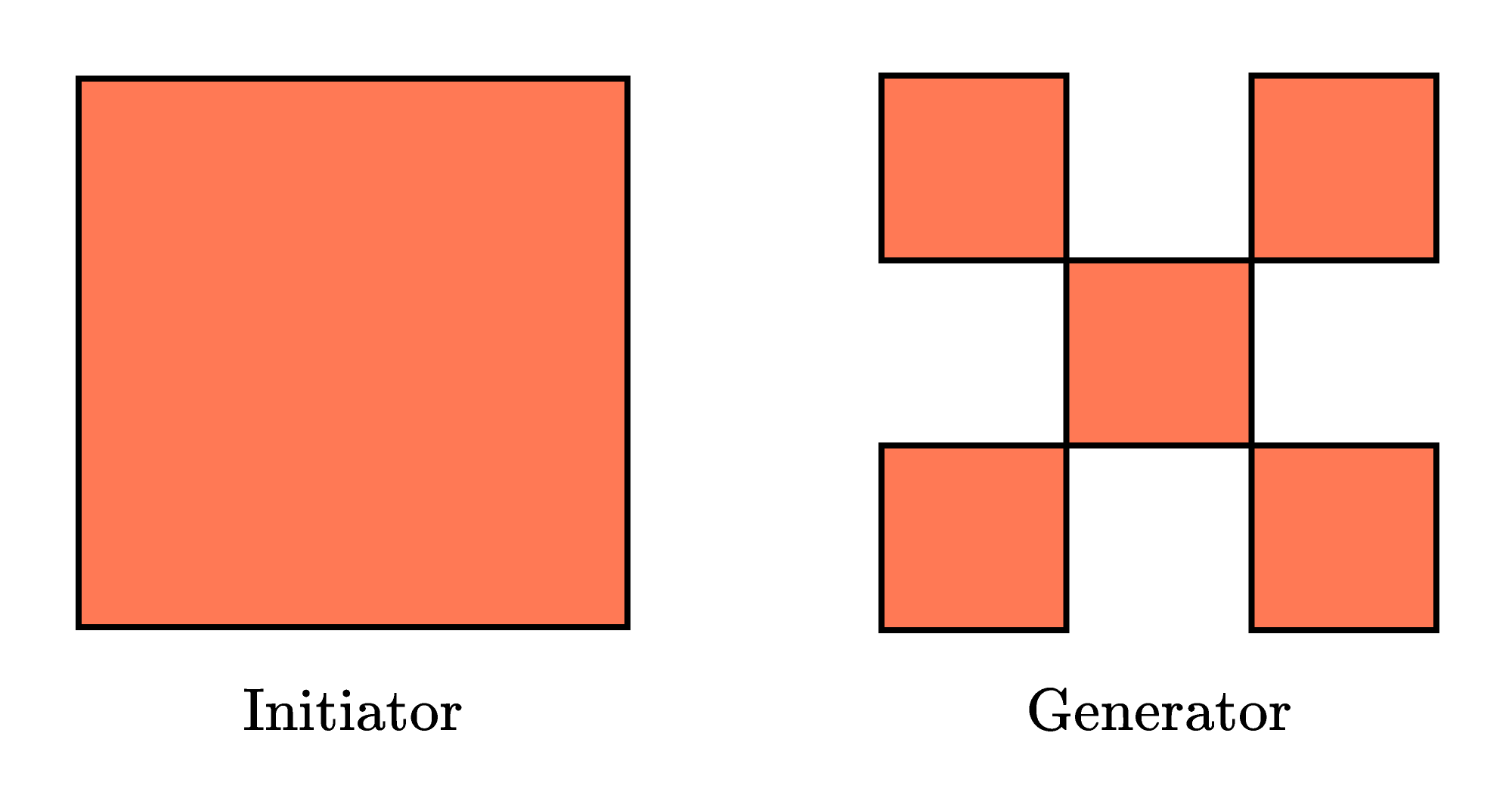To go from one stage to the next, replace every copy of the initiator with a copy of the generator, scaled down as necessary.

The initiator and the generator are the first two stages. The next two look like this: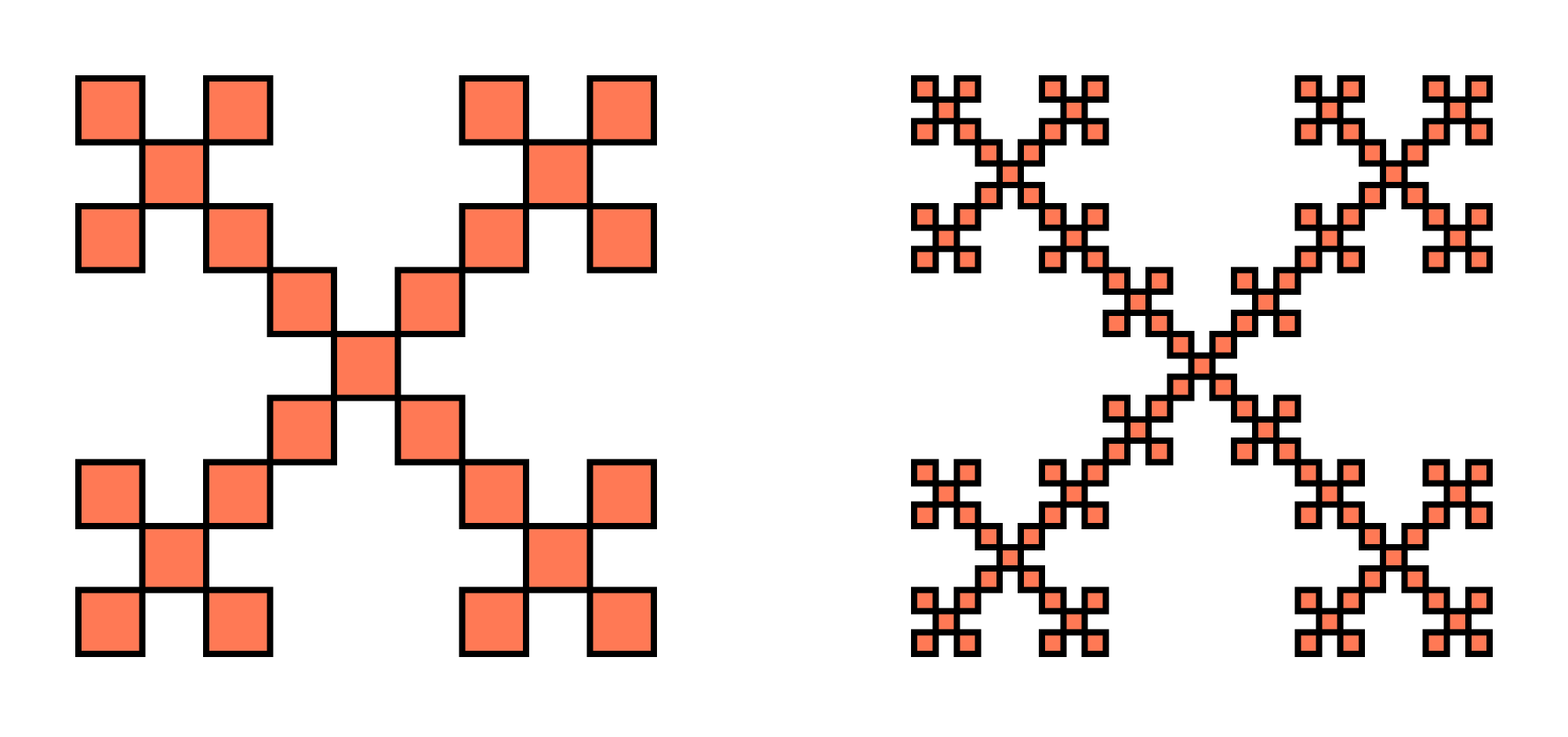The procedure can be repeated ad infinitum.

The dimension, $D,$ of an iteratively defined fractal satisfies the equation $C=S^D$ if, to go from one stage to the next, we replace each initiator with $C$ copies of itself, which have been scaled down by a factor of $S.$

What is the dimension of the fractal defined by this initiator and generator? $\text{ }\\[1em]$